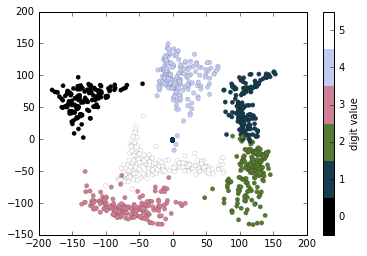# 8.10 自定义颜色条

``````import matplotlib.pyplot as plt
plt.style.use('classic')

%matplotlib inline
import numpy as np
``````

``````x = np.linspace(0, 10, 1000)
I = np.sin(x) * np.cos(x[:, np.newaxis])

plt.imshow(I)
plt.colorbar();
``````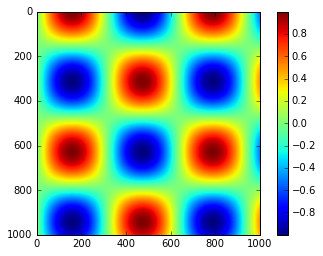## 自定义颜色条

``````plt.imshow(I, cmap='gray');
````````````plt.cm.<TAB>
``````

### 选择颜色表

• 顺序颜色表：它们由连续的颜色序列组成（例如，`binary``viridis`）。
• 发散颜色表：这些通常包含两种不同的颜色，显示相对均值的正偏差和负偏差（例如，`RdBu``PuOr`）。
• 定性颜色表：这些混合颜色没有特定的顺序（例如，`rainbow``jet`）。

`jet`颜色表是 2.0 版之前 Matplotlib 中的默认值，是定性颜色表的一个示例。它的默认状态非常不幸，因为对于表示定量数据来讲，定性映射往往是不良选择。问题之一是，定性映射通常在尺度增加时不显示任何均匀的亮度变化。

``````from matplotlib.colors import LinearSegmentedColormap

def grayscale_cmap(cmap):
"""返回给定颜色表的灰度版本"""
cmap = plt.cm.get_cmap(cmap)
colors = cmap(np.arange(cmap.N))

# 将 RGBA 转换为感知灰度亮度
# cf. http://alienryderflex.com/hsp.html
RGB_weight = [0.299, 0.587, 0.114]
luminance = np.sqrt(np.dot(colors[:, :3] ** 2, RGB_weight))
colors[:, :3] = luminance[:, np.newaxis]

return LinearSegmentedColormap.from_list(cmap.name + "_gray", colors, cmap.N)

def view_colormap(cmap):
"""使用颜色表的灰度等价物来绘制它"""
cmap = plt.cm.get_cmap(cmap)
colors = cmap(np.arange(cmap.N))

cmap = grayscale_cmap(cmap)
grayscale = cmap(np.arange(cmap.N))

fig, ax = plt.subplots(2, figsize=(6, 2),
subplot_kw=dict(xticks=[], yticks=[]))
ax.imshow([colors], extent=[0, 10, 0, 1])
ax.imshow([grayscale], extent=[0, 10, 0, 1])

view_colormap('jet')
``````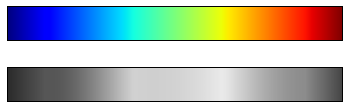``````view_colormap('viridis')
````````````view_colormap('cubehelix')
``````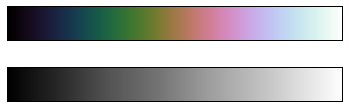``````view_colormap('RdBu')
``````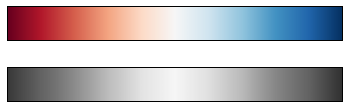Matplotlib 中有大量的颜色表；要查看它们的列表，可以使用 IPython 来探索`plt.cm`子模块。对于在 Python 中使用颜色的更加合乎正道的途径，你可以参考 Seaborn 库中的工具和文档（参见“使用 Seaborn 进行可视化”）。

### 颜色限制和扩展

Matplotlib 允许定制大范围的颜色条。颜色条本身只是`plt.Axes`的一个实例，所以我们学到的所有轴域和刻度的格式化技巧都适用。颜色条有一些有趣的灵活性：例如，我们可以缩小颜色限制，并通过设置`extend`属性，在顶部和底部用三角形箭头指示越界值。这可能会派上用场，例如，如果显示受噪声影响的图像：

``````# 为 1% 的图像像素生成噪声
speckles = (np.random.random(I.shape) < 0.01)
I[speckles] = np.random.normal(0, 3, np.count_nonzero(speckles))

plt.figure(figsize=(10, 3.5))

plt.subplot(1, 2, 1)
plt.imshow(I, cmap='RdBu')
plt.colorbar()

plt.subplot(1, 2, 2)
plt.imshow(I, cmap='RdBu')
plt.colorbar(extend='both')
plt.clim(-1, 1);
``````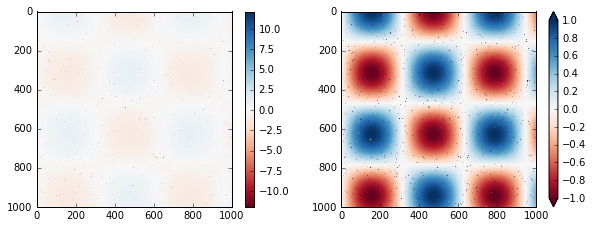### 离散颜色条

``````plt.imshow(I, cmap=plt.cm.get_cmap('Blues', 6))
plt.colorbar()
plt.clim(-1, 1);
``````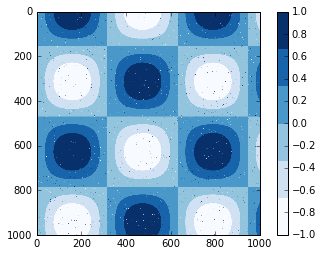## 示例：手写数字

``````# 加载数字 0~5 的图像
# 可视化它们中的几个

fig, ax = plt.subplots(8, 8, figsize=(6, 6))
for i, axi in enumerate(ax.flat):
axi.imshow(digits.images[i], cmap='binary')
axi.set(xticks=[], yticks=[])
``````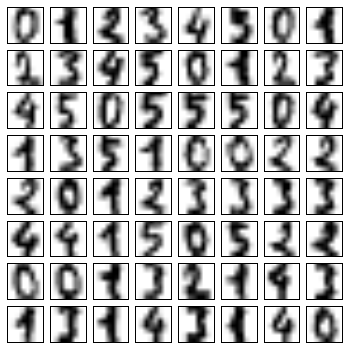``````# 使用 IsoMap 将数字投影到二维
from sklearn.manifold import Isomap
iso = Isomap(n_components=2)
projection = iso.fit_transform(digits.data)
``````

``````# 绘制结果
plt.scatter(projection[:, 0], projection[:, 1], lw=0.1,
c=digits.target, cmap=plt.cm.get_cmap('cubehelix', 6))
plt.colorbar(ticks=range(6), label='digit value')
plt.clim(-0.5, 5.5)
``````